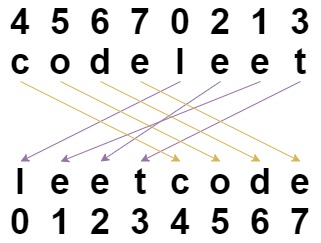# 重新排列字符串

## 1528. 重新排列字符串 (Easy)```输入：s = "codeleet", `indices` = [4,5,6,7,0,2,1,3]

```

```输入：s = "abc", `indices` = [0,1,2]

```

```输入：s = "aiohn", `indices` = [3,1,4,2,0]

```

```输入：s = "aaiougrt", `indices` = [4,0,2,6,7,3,1,5]

```

```输入：s = "art", `indices` = [1,0,2]

```

• `s.length == indices.length == n`
• `1 <= n <= 100`
• `s` 仅包含小写英文字母。
• `0 <= indices[i] < n`
• `indices` 的所有的值都是唯一的（也就是说，`indices` 是整数 `0``n - 1` 形成的一组排列）。

[排序]